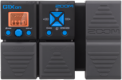# Analisis

Discussion in 'Zoom G1on/G1Xon' started by MatthewWehttam, Nov 9, 2020.

1. AnalisisDevice: Zoom G1Xon
Firmware: 1.21

Name on device: Analisis
Optimized for: Phones/Speaker

Effects chain:Effect: "DZ DRIVE" (Amp simulators), active - "yes"
"Gain" = 40
"Tube" = 0
"Level" = 90
"Trebl" = 60
"Middl" = 50
"Bass" = 40
"Prese" = 60
"CAB" = REVO-1 4x12
"OUT" = LINE

Effect: "ParaEQ" (Dynamics / Filter), active - "yes"
"Freq1" = 40Hz
"Q1" = 2
"Gain1" = -12
"Freq2" = 40Hz
"Q2" = 2
"Gain2" = -12
"Level" = 100

Effect: "ParaEQ" (Dynamics / Filter), active - "yes"
"Freq1" = 6.3kHz
"Q1" = 8
"Gain1" = -12
"Freq2" = 800Hz
"Q2" = 1
"Gain2" = 2
"Level" = 100

Effect: "GraphicEQ" (Dynamics / Filter), active - "yes"
"160Hz" = 0
"400Hz" = 0
"800Hz" = 0
"3.2kHz" = 0
"6.4kHz" = -12
"12kHz" = -12
"Level" = 100
Patch Volume: 100

Note: This is a patch file, you will need to download and install the ToneLib-Zoom software to use the patch.

#### Attached Files:

• ###### Analisis.G1Xon
File size:
396 bytes
Views:
51
Last edited: Nov 10, 2020